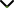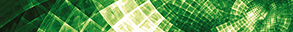# Linear and Nonlinear Matrix Equations: Solutions, Convergence, Perturbation, and Optimization

Publishing date
01 May 2022
Status
Closed
17 Dec 2021

Guest Editors

1Vellore Institute of Technology, Vellore, India

2Shumen University, Shumen, Bulgaria

3Institute for Advanced Studies in Basic Sciences, Zanjan, Iran

This issue is now closed for submissions.
More articles will be published in the near future.

# Linear and Nonlinear Matrix Equations: Solutions, Convergence, Perturbation, and Optimization

This issue is now closed for submissions.
More articles will be published in the near future.

## DescriptionThe study of numerical methods for linear and nonlinear algebraic systems arises when modeling many different problems in applied science. To be more precise, the study of nonlinear matrix equations (NMEs) first arose in the literature with algebraic Riccati equations and plays a central role in several engineering and mathematical models. NMEs have great importance in infinite-horizon optimal control problems in continuous-time or discrete-time formats.

As such, they have been studied extensively in recent decades, producing a vast literature with applications in control theory, dynamical programming, ladder networks, queueing theory, and statistics, among others. Over the years, many authors have studied these types of matrix equations to compute their positive definite solutions (PDS) using different iterative methods, allowing them to identify the solution. Researchers have introduced algorithms for obtaining the PDS of some matrix equations, some of which use the basic fixed point iterative method and its accelerated version, while others use the inversion-free method, two-sided iterative method, Newton's method, monotone Newton-like method, or perturbation bounds of positive solution.

This Special Issue is devoted to publishing modern and noteworthy research on linear and nonlinear matrix equations and all their characteristics. Our objectives are to highlight the latest progress and developments on the theory, techniques, and results of linear and nonlinear matrix equations.

Potential topics include but are not limited to the following:

• Iterative solutions of matrix equations
• Positive definite solutions of matrix equations
• Closed-form solutions and solvability of matrix equations
• Perturbation analysis of matrix equations
• Algebraic matrix equations
• Differential matrix equations
• Numerical algorithms
• Iterative methods with and without memory
• Applications of fixed-point theorems to matrix equations
• Generalized inverses and fast algorithms for matrix functions
• Iterative methods for inverse problems
• Matrix equations with applications to control theory
• Matrix inequalities
• Matrix methods in partial differential equations (PDEs)Journal metrics
See full report
Acceptance rate41%
Submission to final decision45 days
Acceptance to publication26 days
CiteScore0.900
Journal Citation Indicator1.000
Impact Factor1.555Author guidelinesEditorial boardDatabases and indexing

Article of the Year Award: Outstanding research contributions of 2021, as selected by our Chief Editors. Read the winning articles.